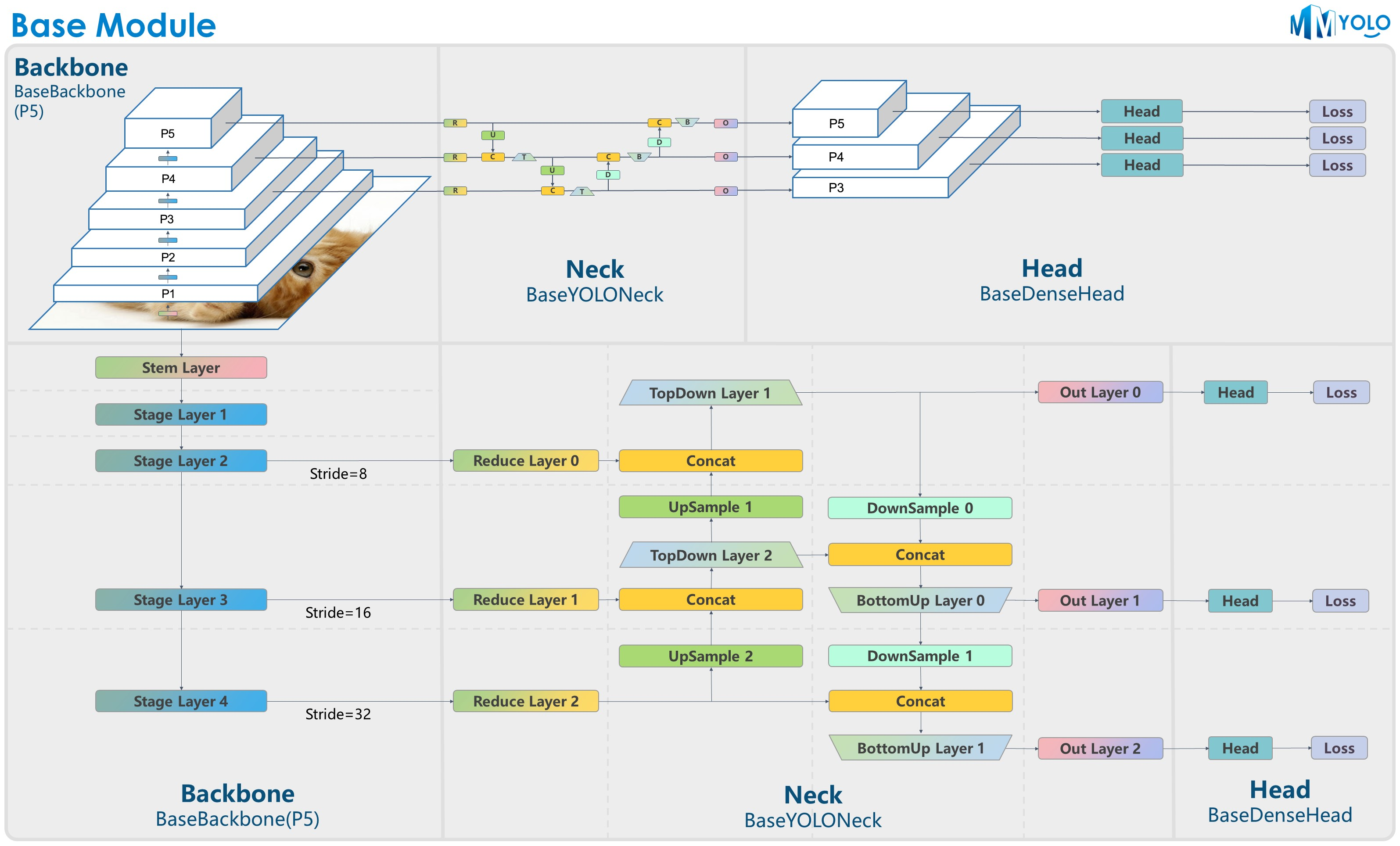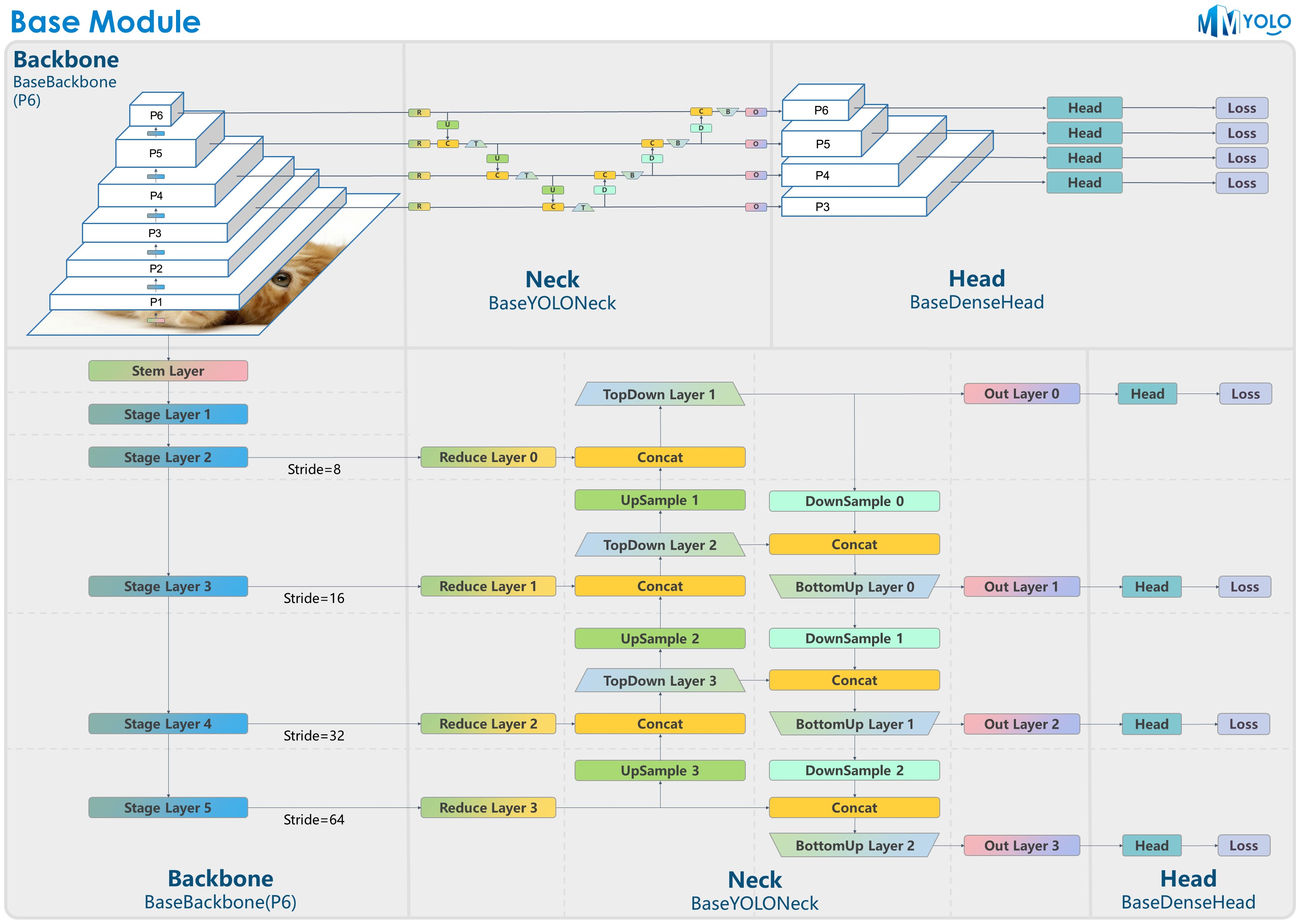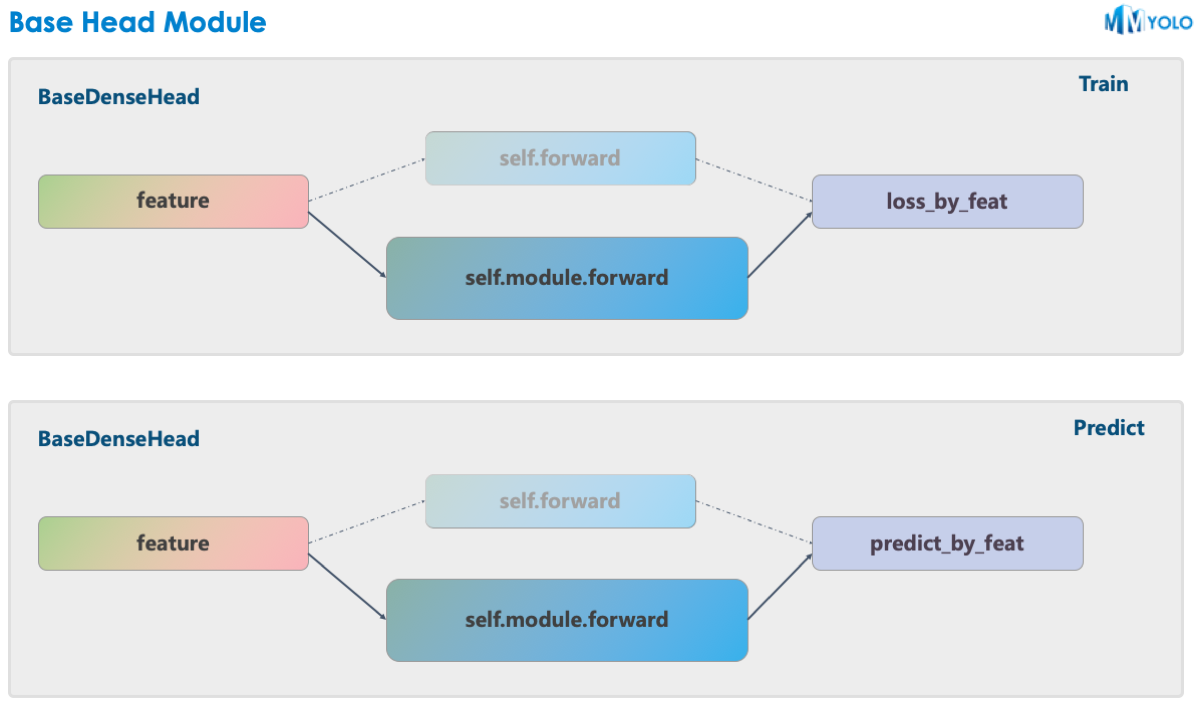Shortcuts

## YOLO series model basic class¶

The structural figure is provided by RangeKing@GitHub. Thank you RangeKing！Figure 1: P5 model structureFigure 2: P6 model structure

Most YOLO series algorithms adopt a unified algorithm-building structure, typically as Darknet + PAFPN. In order to let users quickly understand the YOLO series algorithm architecture, we deliberately designed the BaseBackbone + BaseYOLONeck structure, as shown in the above figure.

The benefits of the abstract BaseBackbone include:

1. Subclasses do not need to be concerned about the forward process. Just build the model as a builder pattern.

2. It can be configured to achieve custom plug-in functions. Users can easily insert some similar attention modules.

3. All subclasses automatically support freezing certain stages and bn functions.

BaseYOLONeck has the same benefits as BaseBackbone.

### BaseBackbone¶

• As shown in Figure 1, for P5, BaseBackbone includes 1 stem layer and 4 stage layers which are similar to the basic structure of ResNet.

• As shown in Figure 2, for P6, BaseBackbone includes 1 stem layer and 5 stage layers. Different backbone network algorithms inherit the BaseBackbone. Users can build each layer of the whole network by implementing customized basic modules through the internal build_xx method.

### BaseYOLONeck¶

We reproduce the YOLO series Neck components in the similar way as the BaseBackbone, and we can mainly divide them into Reduce layer, UpSample layer, TopDown layer, DownSample layer, BottomUP layer and output convolution layer. Each layer can be customized its internal construction by the inheritance and rewrite from the build_xx method.

MMYOLO uses the BaseDenseHead designed in MMDetection as the base class of the Head structure. Take YOLOv5 as an example, the forward function of its HeadModule replaces the original forward method.As shown in the above graph, the solid line is the implementation in MMYOLO, whereas the original implementation in MMDetection is shown in the dotted line. MMYOLO has the following advantages over the original implementation:

1. In MMDetection, bbox_head is split into three large components: assigner + box coder + sampler. But because the transfer between these three components is universal, it is necessary to encapsulate additional objects. With the unification in MMYOLO, users do not need to separate them. The advantages of not deliberately forcing the division of the three components are: data encapsulation of internal data is no longer required, code logic is simplified, and the difficulty of community use and algorithm reproduction is reduced.

2. MMYOLO is Faster. When users customize the implementation algorithm, they can deeply optimize part of the code without relying on the original framework.

In general, with the partly decoupled model + loss_by_feat part in MMYOLO, users can construct any model with any loss_by_feat by modifying the configuration. For example, applying the loss_by_feat of YOLOX to the YOLOv5 model, etc.

Take the YOLOX configuration in MMDetection as an example, the Head module configuration is written as follows:

bbox_head=dict(
num_classes=80,
in_channels=128,
feat_channels=128,
stacked_convs=2,
strides=(8, 16, 32),
use_depthwise=False,
norm_cfg=dict(type='BN', momentum=0.03, eps=0.001),
act_cfg=dict(type='Swish'),
...
loss_obj=dict(
type='CrossEntropyLoss',
use_sigmoid=True,
reduction='sum',
loss_weight=1.0),
loss_l1=dict(type='L1Loss', reduction='sum', loss_weight=1.0)),


For the head_module in MMYOLO, the new configuration is written as follows:

bbox_head=dict(
num_classes=80,
in_channels=256,
feat_channels=256,
widen_factor=widen_factor,
stacked_convs=2,
featmap_strides=(8, 16, 32),
use_depthwise=False,
norm_cfg=dict(type='BN', momentum=0.03, eps=0.001),
act_cfg=dict(type='SiLU', inplace=True),
),
...
loss_obj=dict(
type='mmdet.CrossEntropyLoss',
use_sigmoid=True,
reduction='sum',
loss_weight=1.0),
loss_bbox_aux=dict(type='mmdet.L1Loss', reduction='sum', loss_weight=1.0)),
train_cfg=dict(
assigner=dict(
type='mmdet.SimOTAAssigner',
iou_calculator=dict(type='mmdet.BboxOverlaps2D'))),


© Copyright 2022, OpenMMLab. Revision 8c4d9dc5.

Built with Sphinx using a theme provided by Read the Docs.